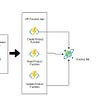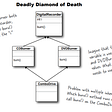# What is DFS ?

Let’s discuss about DFS first. DFS means Depth First Search. It is a searching algorithm in a graph data structure. If you are from a Computer Science background, you have heard that before. It is an easy algorithm and must know algorithm to learn for a Programmer or any other Computer Science field. The search starts from the root node. Then it looks for its neighboring nodes. Then it chooses a neighbor node that is not yet visited, finally we move to the chosen node.

Whenever a node is visited, the node is pushed to a stack data structure which stores the path we are currently on. If a node has no neighbors, then we pop the last item from the stack and consider that node as the current node. Now repeat the same process until the stack becomes empty.

For the graph above, the DFS output will be as follows:

1 -> 2 -> 4 -> 7 -> 5 -> 3 -> 6 -> 8

That’s it! Let’s discuss about the maze creation.

# Maze Creation

Let’s start creating the maze with the algorithm we learned right now. I am going to implement the simplest way possible to understand. Let’s go through the pseudo code first.

`// for simplicity stack can be implemented as an arraystack = []// take the initial node and mark it as visited, add it to the stackstart.visited = Truestack.append(start)// do the operation until the stack become emptywhile len(stack) > 0:    //pop the last node from the stack and make it as current    current = stack.pop(0)    if current.has_unvisited_neighbors():        // current node has unvisited neighbors get a random one        neighbor = current.get_random_neighbor()        // push the current node to stack        stack.append(current)        // remove the wall between the current and neighboring node        remove_wall_between(current, neighbor)        // moving to neighboring node and adding it to stack        neighbor.visited = True        stack.append(neighbor)`

Let’s create the game as a web app do that we can visualize the algorithm. I am just going to go through the code that corresponds to algorithm, you can implement the app as per your wish. First, let’s see the structure for each node. You can use the class object to the make a node but for simplicity sake, I have made it as a simple object. It is created as follow:

`{     row: i,     col: j,     // borders     top: true,     left: true,     bottom: true,     right: true,     // check if already visited     visited: false}`

The top, left, right, bottom values implies the border of each node. The row and col represents the actual row and col of the node. Visited simply used to track whether the node is already visited or not. Now, let’s get into creating maze.

`export function createMaze(grid) { // stack DS to track the current node const stack = []// starting with first node and mark it as visited stack.push(grid) grid.visited = truewhile (stack.length > 0) {  // taking the last item visited in the stack and get all their nighbours  const current = stack.pop()  const neighbours = getAllNeighbours(current, grid)// proceed only if there is atleast one neighbour  if (neighbours.length > 0) {   // as the current node has a nighbour it is added to the stack   stack.push(current)   // get a radom neighbour from the neighbours list   const neighbour = getRandomNeighbour(neighbours)   removeWall(current, neighbour)// mark the neighbour as visited   neighbour.visited = true   stack.push(neighbour)  } }}`

To get the neighboring nodes, we can separate that in another function as follows:

`// get all the neighbour for maze creation// visited neighbour is not valid herefunction getAllNeighbours(current, grid) { const neighbours = [] const [row, col ] = getPos(current)if (row > 0 && !grid[row - 1][col].visited)// top  neighbours.push(grid[row - 1][col])if (row < ROWS - 1 && !grid[row + 1][col].visited)// bottom  neighbours.push(grid[row + 1][col])  if (col > 0 && !grid[row][col - 1].visited)// left  neighbours.push(grid[row][col - 1])  if (col < COLS - 1 && !grid[row][col + 1].visited)// right  neighbours.push(grid[row][col + 1])return neighbours}`

and for removing the wall:

`removeWall(current, neighbour) {// removing the wall between the current and the considered neighbour   if (current.row > neighbour.row) {    current.top = false    neighbour.bottom = false   }   else if (current.row < neighbour.row) {    current.bottom = false    neighbour.top = false   }   else if (current.col > neighbour.col) {    current.left = false    neighbour.right = false   }   else {    current.right = false    neighbour.left = false   }}`

Considering that each node has the border on all the sides. So if we want to remove the wall between current and neighbor node, we need to remove the wall from both the nodes. For example, if the current is below the neighboring node, then we need to remove the top border of the current and bottom border of the neighbor node. To get a random neighbor we use another function as follows:

`// get a random neighbour from the list of neighboursfunction getRandomNeighbour(neighbours) { const index = Math.floor(Math.random() * neighbours.length) return neighbours[index]}`

If you are interested in viewing the whole project (which is damn so simple project), checkout the code in GitHub. If you just wanna try the app to be in action, you can check that out here. In this project, I have also made functions to find the path between any start and end node using an algorithm called A* path finding algorithm. It was really a great algorithm to learn. If you are interested, I have already made an article about that. You can check that out here -> https://18ganapathy04.medium.com/a-path-finding-algorithm-1dd35f49c164

--

--

--

## More from Ganapathy P T

Web Developer all the way from India

Love podcasts or audiobooks? Learn on the go with our new app.

## Recommended from Medium## How to Deploy Your Vue App to Heroku to Boost Your Productivity## Azure Functions — Creating a serverless API with NodeJS## Subsequence of a String in(Java) Iterative method## Styling SVG icon in React.js## Refactoring Your Component with React Hooks## Production Problems Kill Confidence in Development Teams## Ganapathy P T

Web Developer all the way from India

## Computer Floating-Point Arithmetic and round-off errors## Chapter 8 — Interfaces and Abstract classes